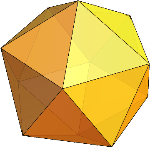Search IntMath
Close

450+ Math Lessons written by Math Professors and Teachers

5 Million+ Students Helped Each Year

1200+ Articles Written by Math Educators and Enthusiasts

Simplifying and Teaching Math for Over 23 Years

# What Is an Icosahedron in Geometry?The icosahedron is one of the five Platonic solids, which are 3D geometric shapes with identical faces and angles. It has 20 faces, 30 edges, and 12 vertices. It is also one of the polyhedra, which are 3D shapes that are made up of flat surfaces.

## History of the Icosahedron

The icosahedron is one of the oldest known geometric shapes. It was first documented by the ancient Greeks in the fourth century BC. It has since been used in many different contexts, including mathematics, architecture, engineering, and art.

The icosahedron has also been used in modern times to create sculptures, jewelry, and other decorative items. It is also a popular choice for creating educational models, as it can easily be constructed from paper or other materials.

## Geometric Properties of the Icosahedron

The icosahedron has 20 faces that are all identical, meaning that all of the angles, edges, and vertices are the same. Each face is a regular triangle, and the icosahedron is made up of 12 vertices, 30 edges, and 20 faces. All of the edges are equal in length and all of the faces are equilateral triangles.

The icosahedron is a symmetrical shape, meaning that if it is cut in half, the two halves will be identical. This symmetry is also true if the icosahedron is rotated in any direction. This makes it an excellent shape for use in mathematics and engineering.

## Applications of the Icosahedron in Mathematics

The icosahedron is a popular choice for use in mathematics, as it is a symmetrical shape that is easily constructed. It can be used to explore the properties of 3D geometry, as well as to help students understand the concept of Platonic solids and polyhedra.

The icosahedron can also be used to explore the concept of symmetry and to understand the relationships between different geometric shapes. It is also often used in art and architecture, as it is a visually appealing shape.

## Practice Problems

1) How many faces does an icosahedron have?
Answer: An icosahedron has 20 faces.

2) What type of geometric shape is an icosahedron?
Answer: An icosahedron is a 3D geometric shape with identical faces and angles.

3) How many edges does an icosahedron have?
Answer: An icosahedron has 30 edges.

4) What type of shape is an icosahedron?
Answer: An icosahedron is a polyhedron, which is a 3D shape made up of flat surfaces.

5) Is an icosahedron a symmetrical shape?
Answer: Yes, an icosahedron is a symmetrical shape.

6) What type of solid is an icosahedron?
Answer: An icosahedron is a Platonic solid.

## Conclusion

The icosahedron is one of the five Platonic solids, which are 3D geometric shapes with identical faces and angles. It has 20 faces, 30 edges, and 12 vertices. It is also one of the polyhedra, which are 3D shapes that are made up of flat surfaces. The icosahedron is a popular choice for use in mathematics, as it is a symmetrical shape that is easily constructed. It is also often used in art and architecture, as it is a visually appealing shape.

## FAQ

### What is an icosahedron?

An icosahedron is a polyhedron with 20 faces. It is a platonic solid, composed of 20 equilateral triangles. It is a regular, convex polyhedron, with 12 vertices and 30 edges.

### What is the surface area of an icosahedron?

The surface area of an icosahedron is equal to 5 times the square of its edge length. The formula is A = 5 * a2, where a is the edge length of the icosahedron.